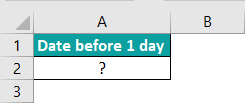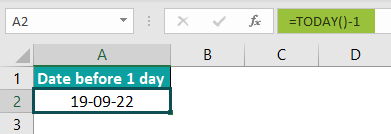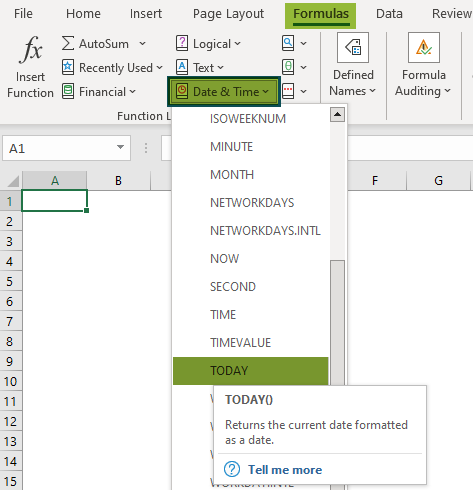# TODAY Excel FunctionArticle byExcelMojo Team## What Is TODAY Function In Excel?

The TODAY function in Excel helps users to calculate the current date. Using this function, we can add or subtract the dates, calculate months and years before or after a certain date, and calculate the age from the birth date.

The TODAY Excel function is an inbuilt function which means that we can enter the function directly in a cell as a formula or insert it from the Formulas tab.

For example, we will apply the TODAY function to calculate today’s date in cell A1.

The TODAY Excel formula entered in cell A1 is =TODAY().

The output is 29 July 2022, as shown in the above image. The function returns the current date.

###### Key Takeaways
• When we use the TODAY Excel function, we will get the output as todays or the current date.
• We can use the following keyboard shortcuts, to enter today’s date in Excel as a static, unchangeable value.
• To Insert Today’s Date CTRL + ;
• To Insert Current Time– CTRL + SHIFT + ;
• To Enter Current Date and Time– CTRL + ; Space CTRL + SHIFT + ;
• For the TODAY function in Excel to give accurate results, the cell should be set from “General” to “Date” Format.
• If the function does not get an updated date, press the F9 key to recalculate and update the value.

### TODAY() Excel Formula

The Syntax of the TODAY function in Excel formula is,

The TODAY Excel Function does not have any arguments.

### How To Use TODAY Excel Function?

We can use the TODAY Excel Function in the following methods.

1. Access from the Excel ribbon.
2. Enter the worksheet manually.

#Method 1 – Access from the Excel ribbon

Choose an empty cell > select the “Formulas” tab > go to the “Function Library” group > click the “Date & Time” drop-down> click on the “Today” function option, as shown below.

The Today() formula is inserted in the cell, and instantly a window called “Function Arguments” appears.

This function does not require any inputs. Therefore, only select “OK”.

#Method 2 – Enter the worksheet manually

1. Select an empty cell for the output.
2. Type =TODAY() in the selected cell. [Alternatively, type “=T” and double-click the TODAY function from the list of suggestions shown by Excel].
3. Press the “Enter” key.

# Basic Example

We will calculate today’s date using Excel’s CONCATENATE(), TEXT(), and TODAY() functions.

In the following table, the data is,

• Column A contains the Date to be calculated.

The steps to calculate the dates using the TODAY Excel function are as follows:

Step 1: Select cell A2 and enter the formula =CONCATENATE(“Today’s Date is ”,TEXT(TODAY(),“mm/dd/yyyy”)).

Step 2: Press the “Enter” key.

The output is “Today’s Date is 07/29/2022”, as shown above.

### Examples

We will understand the TODAY function in Excel format with some advanced scenarios.

#### #1 Example

We will calculate today’s date, one day before today, and one day after today using the TODAY function in Excel format.

In the following table, the data is,

• Column A contains the Details.
• Column B contains the Date to be calculated.

The steps to calculate the dates using the TODAY in Excel are as follows:

Step 1: Enter the formulas =TODAY()-1, =TOTAL(), and =TOTAL()+1 in the cells B2, B3, and B4 respectively.

Step 2: Press the “Enter” key every time the formula is entered.

The output is shown above. The formulas are displayed in column C for our reference.

#### #2 Example

We will calculate age from birth using Excel’s YEAR() and TODAY() functions.

In the following table, the data is,

• Column A contains the Year of Birth.
• Column B contains the Age to be calculated.

The steps to calculate the age using the TODAY Excel function are as follows:

Step 1: Select cell B2 and enter the formula =YEAR(TOTAL())-A2.

Step 2: Press the “Enter” key. The output is shown below.

Step 3: Drag the formula from cell B2, using the fill handle, to cell B5.

The output is shown above.

#### #3 Example

We will highlight today’s date using the Conditional Formatting method.

In the following table, the data is,

• Column A contains the list of Dates.

The steps to highlight today’s date using the Conditional Formatting method are as follows:

Step 1: Select the dates in cell range A2:A8, go to the “Home” tab > go to the “Styles” group > click the “Conditional Formatting” drop-down > select the “Manage Rules…”, as shown below.

Step 2: The “Conditional Formatting Rules Manager” window opens. Now, select the “This Worksheet” option in the “Show formatting rules for:” drop-down, and click the “New Rule…” button.

Step 3: The “New Formatting Rule” window opens.

• First, select the “Use a formula to determine which cells to format” option from the “Select a Rule Type:” group.
• Next, in the “Edit the Rule Description:” group, enter the formula =\$A2=TODAY() in the “Format values where this formula is true” field.
• Finally, click on the “Format” button.

Step 4: The “Format Cells” window opens. Here, select the “Fill” tab > go to the “Background Color” group, and select the desired color; here, “Yellow” > click “OK” to close the “Format Cells” window.

Step 5: Click “OK” to close the “New Formatting Rule” window.

Step 6: Click “OK” to close the “Conditional Formatting Rules Manager” window.

The output is shown below. Today’s date is highlighted in yellow.

### Important Things To Note

• The TODAY Excel function is volatile because every time we open a worksheet, it updates itself continuously.
• Also, the “Automatic” option must be selected for the TODAY Excel function to update automatically. Therefore, to turn it on, go to the “Formulas” tab > the “Calculation” group >click the “Calculation Options” drop-down > select the “Automatic” option, as shown below.
• We can type the TODAY function in Excel formula in the output cell as =T [in older Excel versions] or =To [in newer Excel versions as many new functions are added] and then double-click the TODAY function from the list of suggestions shown by Excel.
• Other than the TODAY() function, the NOW() function is also used to enter the current date and time.

Why is the TODAY function not working in Excel?

The TODAY function in Excel might not work due to the following reasons;

1. The entered syntax of the TODAY() function may be incorrect.
2. Extra spaces in between the formula of the TODAY() function.
3. The cell format should be Date, either long or Short Date, but not any other format.
4. Automatic recalculation might not be selected in Excel.

How to Insert TODAY function in Excel?

The simple and easy way to Insert TODAY function in Excel is,

1. Select an empty cell for calculating the Date.
2. Type =TODAY() in the selected cell. Alternatively, type =T or =To and double-click on the TODAY function from the list of suggestions shown by Excel.
Press the “Enter” key.

For example, we apply the TODAY function to calculate the date before one day in cell A2.The TODAY function in Excel formula entered is =TODAY()-1, and the result is “28-07-2022”.

To set the cell format,

1. Go to the “Home” tab > go to the “Number” group > click on the “Number Format” drop-down > select the “More Number Formats…” option.
2. The “Format Cells” window opens. Here, select the “Number” tab > and click the “Date” option on the left > click “OK”.

We will get the output in the date format, as shown below.Where is the TODAY function in Excel?

We can use the TODAY function in Excel as follows:

First, choose an empty cell > select the “Formulas” tab > go to the “Function Library” group > click the “Date & Time” drop-down > click on the “Today” function option, as shown below.